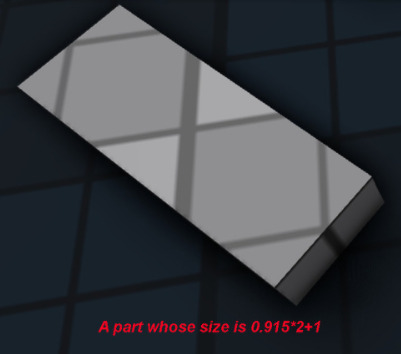# I also how to be able to find the length of a slope that’s rotated any degrees not just 45 degrees. I need the equation.

I literally do not know any mathematical terms of how to explain this, but basically I’m trying to find out look, if you have a vector that’s (1,-1) and 45 degrees

-1.001
1.001
is close, but still not there.

0.915 is what I found and I multiplied it by 2 and add it by +1 and made it the part’s X size. I want the end point to be on the grid of 1 stud.

# I also how to be able to find the length of a slope that’s rotated any degrees not just 45 degrees. I need the equation.

3 Likes

Alright guys allow me to rephrase. I want the end point of any slope or rotated part to be on a 1x1 grid.

But I do not know what to set the size of the part or the equation for it. I just know a number 0.915 that I obtained from bruteforcing

If you guys want a rblx file here it is
smarta.rbxm (4.2 KB)

1 Like

It’s pretty hard to understand what you’re trying to achieve or what value you are looking for. In one part you ask where vectors intersect and yet I don’t see how that applies to what you’re doing or the examples you’ve given. Could you try to explain the issue you have in a more clear way? Is it that you want a part to fit a 1x1 grid? What are you trying to achieve?

Is the part with size 1 (1,1,1) or? The grid you’ve shown is a 2x2 if it’s the standard Roblox baseplate.

I’m trying to make the end points of a part or a linear slope on roblox align to a 1x1 grid. But when it is the length of 1 stud it does not align to the 1x1 grid. Read what I said above. I’m not very good at mathematical terminology so I don’t know what to call these things but I made some pictures and you can view them See the thing on the right?

I want the red dots to be where those white lines are. The brick is 1 stud long. If I make it 1.54119616746902 studs long which is the magnitude of the position of the brick to the white line, it won’t be correct, because the magnitude of the vector (-1,1) is 1.414213562, or something. I don’t know what I’m talking about and I’m way in over my head. Can you please call someone you know that’s good at math here

I need math experts here please, people who know high school geometry, algebra, calculus, trigonometry, linear algebra, and programming

Can’t you just use the Pythagorean theorem to solve the problem?

Judging by this image:It seems though that a 2 by 2 stud required a part with the length of `2.83` studs. Which, not coincidently, is the same value you get when you punch in those numbers in the formula (rounded to the closest 0.01):

To get the length of the part, simply run the Pythagorean theorem. The `a` leg would be the `x` axis in studs, and the `b` leg will be the `z` axis in studs.

To get the rotation, you can use this code snippet:

``````local function GetRotation(xLength, zLength)
return math.atan2(zLength, xLength) * 180 / math.pi
end
``````
1 Like

Hi!
Sorry for my English, I use a translator
Hmm… If look at your images, you can understand that the red dots should be on the graph in integer coordinates.
From that, I came up with the idea of simply getting neighboring points from the cframe object, and then rounding them:

``````math.floor()--- for negative
math.round()--- for positive
``````

And then position the object between them and count the distance between one of them for setting size.

At least this is just an idea to solve your problem.robloxapp-20210626-0538359.wmv (2.0 MB)

``````Xoriginal = workspace.Part.Size.X
while wait() do
right= workspace.Part.CFrame * CFrame.new( Xoriginal,0,0)
left = workspace.Part.CFrame * CFrame.new(-Xoriginal,0,0)
left = CFrame.new(math.floor(left.X),math.floor(left.Y),math.floor(left.Z))
right = CFrame.new(math.round(right.X),math.round(right.Y),math.round(right.Z))
workspace.Part.Position = right:Lerp(left,0.5).Position
workspace.Part.Size =Vector3.new((workspace.Part.Position-right.Position).Magnitude*2,
workspace.Part.Size.Y,
workspace.Part.Size.Z)
end

``````

It sounds like you want the Hypotenuse (the diagonal long length, or slope as you described it). You definitely don’t need calculus. You can use Euclid’s 47th Proposition, or the pythagorean theorem - which someone else has described in adequate detail.